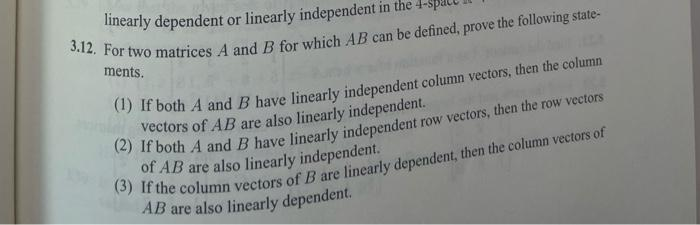# Question 3.12 please linearly dependent or linearly independent in the 3.12. For two matrices A and B for which AB can be defined, prove the following state- ments. (1) If both A and B have linearly independent column vectors, then the column Vectors of AB are also linearly independent. 2) If both A and B have linearly independent row vectors, then the row vectors of AB are also linearly independent. (3) If the column vectors of B are linearly dependent, then the column vectors of AB are also linearly dependent